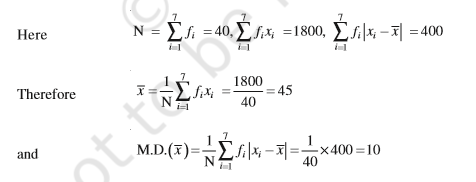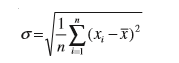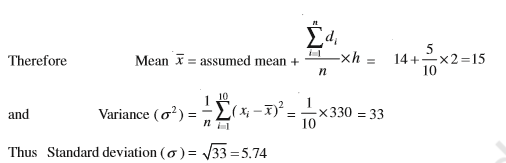or# Statistics NCERT Solutions : Class 11 Maths

## NCERT Solutions for Class 11 Maths : StatisticsFilter
Filters :
Classes
•
•
•
•
•
•
•
Chapters
•
•
•
•
•
•
•
•
•
•
• 6 More
Exercises
•
•
•
•
•

### NCERT Class 11 | STATISTICS | Miscellaneous Exercise | Question No. 04

Given that ` bar x` is the mean and `sigma^2` is the variance of n observations `x_1x_2` , ..., `x_n` . Prove that the mean and variance of the observations `a x_1,""""a x_2` , `a x_3,""""dot""""dot""""dot"""",""a x_n` are `a bar x` and `a^2sigma^2` ,### NCERT Class 11 | STATISTICS | Miscellaneous Exercise | Question No. 05

The mean and standard deviation of 20 observations are found to be 10 and 2, respectively. One rechecking, it was found that an observation 8 was incorrect. Calculate the correct mean and standard deviation in each of the following cases. (i)      If wrong item is omitted (ii)     If it is replaced by 12.### NCERT Class 11 | STATISTICS | Miscellaneous Exercise | Question No. 06

The mean and standard deviation of marks obtained by 50 students of a class in three subject, Mathematics, Physics and Chemistry are given below: Subject &nbsp;&nbsp;&nbsp;&nbsp; Mathematics&nbsp;&nbsp;&nbsp;&nbsp;&nbsp;&nbsp;&nbsp;&nbsp; Physics &nbsp;&nbsp;&nbsp;&nbsp;&nbsp;&nbsp;&nbsp;&nbsp;&nbsp;&nbsp;&nbsp;&nbsp;&nbsp;&nbsp;&nbsp;Chemistry&nbsp;&nbsp;&nbsp; &nbsp;&nbsp;&nbsp;&nbsp;&nbsp;&nbsp;&nbsp;&nbsp;&nbsp;&nbsp;&nbsp;&nbsp;&nbsp;&nbsp;&nbsp;&nbsp;&nbsp;&nbsp;&nbsp;&nbsp;&nbsp;&nbsp; Mean&nbsp;&nbsp; &nbsp;&nbsp;&nbsp;&nbsp;&nbsp;&nbsp;&nbsp;&nbsp; 42&nbsp;&nbsp;&nbsp;&nbsp;&nbsp;&nbsp;&nbsp;&nbsp;&nbsp;&nbsp;&nbsp;&nbsp;&nbsp;&nbsp;&nbsp;&nbsp;&nbsp;&nbsp;&nbsp;&nbsp;&nbsp;&nbsp;&nbsp;&nbsp;&nbsp;&nbsp;&nbsp;&nbsp; 32&nbsp;&nbsp;&nbsp;&nbsp;&nbsp;&nbsp;&nbsp;&nbsp;&nbsp;&nbsp;&nbsp;&nbsp;&nbsp;&nbsp;&nbsp;&nbsp;&nbsp;&nbsp;&nbsp;&nbsp;&nbsp;&nbsp;&nbsp;&nbsp;&nbsp; 40.9 Standard&nbsp;&nbsp; &nbsp;&nbsp;&nbsp; 12&nbsp;&nbsp;&nbsp;&nbsp;&nbsp;&nbsp;&nbsp;&nbsp;&nbsp;&nbsp;&nbsp;&nbsp;&nbsp;&nbsp;&nbsp;&nbsp;&nbsp;&nbsp;&nbsp;&nbsp;&nbsp;&nbsp;&nbsp;&nbsp;&nbsp;&nbsp;&nbsp; 15&nbsp;&nbsp;&nbsp;&nbsp;&nbsp;&nbsp;&nbsp;&nbsp;&nbsp;&nbsp;&nbsp;&nbsp;&nbsp;&nbsp;&nbsp;&nbsp;&nbsp;&nbsp;&nbsp;&nbsp;&nbsp;&nbsp;&nbsp;&nbsp;&nbsp; 20 Deviation Which of the three subjects shows the highest variability in marks and which shows the lowest?### NCERT Class 11 | STATISTICS | Solved Examples | Question No. 13

Two plants A and B of a factory show following results about the number of workers and the wages paid to them.                                                       A                                  B No. of workers                              5000                      6000 Average monthly wages               Rs 2500                 Rs 2500 Variance of distribution                 81                                100  of wages In which plant, A or B is there greater variability in individual wages?Doubtnut one of the best online education platform provides free NCERT Solutions of Maths for Class 11 Statistics which are solved by our maths experts as per the NCERT (CBSE) guidelines. We provide the solutions as video solutions in which concepts are also explained along with answer which will help students while learning , preparing for exams and in doing homework. These Solutions will help to revise complete syllabus and score more marks in examinations.

Get here free, accurate and comprehensive NCERT Solutions for Class 11 Maths Statistics which have been reviewed by our maths counsellors as per the latest edition following up the CBSE guidelines. We provide video solutions in which solutions to all the questions of NCERT Class 11 Maths textbook are explained in step by step and detailed way.

## The Topics of the Chapter Statistics are :

VARIANCE & STANDARD DEVIATIONS

MEASURE OF DISPERSION

It contains these exercises along with solved examples. All the exercises are solved in the video. Select the exercise to view the solutions exercisewise of the Chapter Statistics:

## NCERT Solutions Class 11 Maths Chapter Statistics Exercises:

We have covered all the exercises and also Solved examples in the videos. Along with the practise exercise Students should also practise solved examples to clear the concepts of the Statistics. If incase you have any doubt you can watch the solutions for the given questions.Watch the solutions for the given questions in which it is also explained in the video steps to solve the questions along with answers.

In this chapter, we will focus on various statistical concepts like measures of dispersion, mean deviation, range, variance and standard deviation, analysis of frequency distributions, etc. There are lots of exercises and examples that have been provided by Doubtnut, which makes it much easier for the students to get the best idea on how to come up with the perfect solution. This makes it even possible to score good marks that make them feel quite confident as well.  Getting a clear idea of how to attempt the different questions effortlessly helps to clear their exams with flying colors.

NCERT textbooks are one of the most referred books of maths that helps thousands of CBSE board students achieve high scores in their exams. NCERT maths class 11 textbooks which are provided to the students help them in clearing their basic concepts. However, the NCERT textbook does not provide a comprehensive solution to all the problems of the chapters covered. The students are not able to get in-depth knowledge that leads to difficulty in attempting some of the questions that are asked in the examinations. To master the subject of maths, one needs to practice different types of problems rigorously. This issue is easily overcome by our e-learning online platform Doubtnut, which presents a detailed solution to each question in the book.

Doubtnut provides video and pdf solutions of each question mentioned in the NCERT maths textbook.  The video tutorials are prepared in an easy to understand language for better comprehension and retention. The video solutions prove to be useful in preparation for competitive exams like JEE Main and Advanced. Learning maths from Doubtnut makes the students quite confident on how to deal with the different problems effortlessly. By following all the right approach, the students can get the ultimate idea of how to tackle the questions swiftly. Therefore, students who are willing to succeed in the subject of maths, as well as other subjects, should definitely try to look forward to the most useful video tutorials. This would never leave any unattended queries. With step by step instructions included in video solutions, it becomes possible for the students to get the clear picture as to how questions are set in the examinations.

## Topics included NCERT Class 11 Maths Solutions Chapter 15 Statistics

### Introduction

It is known that with the help of analysis and interpretation, it is possible to make decisions about the data. Finding a representative value for a given set of data has been discussed previously. So, here, we will start from the topic related to measures of dispersion. We will learn about the methods of measures of dispersion and also look forward to their way of calculation for grouped and ungrouped data.

### Measures of Dispersion

You should understand that the dispersion in data is measured on the types of the measure of central tendency along with the observations. The different types of measures of dispersion include range, quartile deviation, mean deviation, and standard deviation. Here, we will discuss all of these measures except the quartile deviation.

### Range

The difference between the maximum and minimum value is known as the Range of the data. So, Range = Maximum value – Minimum value. The range of data provides us with a rough idea of scatter or variability but at the same time, but it does not give any details regarding the dispersion of the data from central tendency measure. Therefore, in order to get a clear idea about it, it needs some other measure of variability. So, mean deviation and standard deviation are the important measures of dispersion where we are going to discuss them in this chapter.

### Mean Deviation

It is to be noted that the sum of the deviations needs to be obtained in order to find the mean. You should also know that there will be negative and positive for some of the deviations. In this case, it is quite important to note that the sum of deviations may vanish.

So, Mean of deviations =      Sum of deviations         =  0 = 0

Number of observations      n

Therefore, regarding the measure of dispersion is concerned; finding the mean of deviation is not useful.

It is important to take the mean of the absolute values of the deviations from the central value which is important to find the measure of dispersion. This is known as the ‘mean deviation.’ This is denoted by ‘a’ which is the mean of the absolute values of the deviations from the central value. Therefore,

M.D. (a) = Sum of absolute values of deviations from ’a

Number of observations

• Mean deviation for ungrouped data: In order to understand how to calculate mean deviation for ungrouped data, we will have a look at an example:

Example: Can you find out the mean deviation about mean for the following data?

12, 6, 7, 4, 13, 12, 10, 8

Solution:  Let us proceed step-wise.

Step 1:  Mean of the given data is

X =  12, 6, 7, 4, 13, 12, 10, 8 = 72 = 9

8                          8

Step 2: The deviations of the respective observations from the mean

­­_             _

x,   i.e. xi-x are

12-9, 6-9, 7-9, 4-9, 13-9,12-9, 10-9,8-9

Or, 3,-3, -2, -5, 4, 3, 1, -1

Step 3: The absolute values of the deviations,i.e.,| xi– x | are

3, 3, 2, 5, 4, 3, 1, 1

Step 4:  The required mean deviation about the mean is

= 3 + 3 + 2 + 5 + 4 + 3 + 1 + 1 = 22 = 2.75

8                             8

• Continuous Frequency Distribution: Here, the classification of data is done with the help of class intervals without gaps and that too with their respective frequencies.
• Below is an example of the continuous frequency distribution for the marks obtained by 100 students.
 Marks Obtained 0 – 10 10 - 20 20 - 30 30 - 40 40 - 50 50 - 60 Number of Students 12 18 27 20 17 6

â€‹â€‹â€‹â€‹â€‹

• Mean deviation about mean: In order to understand this, we will have a look at an example below:

Example:  Can you find the mean deviation about the mean for the following data?

 Marks Obtained 10 –20 20 - 30 30 - 40 40 - 50 50 - 60 60 –70 70-80 Number of Students 2 3 8 14 8 3 2

 Marks obtained Number of Students       fi Mid- Points        xi fixi __ | xi – x | __ fi | xi – x | 10 – 20   20 – 30   30 – 40   40 – 50   50 – 60   60 – 70   70 - 80 2       3       8      14        8        3         2 15       25       35       45       55       65       75 30       75      280      630      440      195      150 30      20      10        0      10      20      30 60      60      80        0      80      60      60 40 1800 400### Standard Deviation

The standard deviation, usually denoted by σ isWe will get a good and clear idea by having a look at an example:

Example:   Can you find the variance of the following data: 10, 18, 20,6, 8, 14, , 12, 16, 22, 24?

 xi di = xi – 14            2 Deviations from mean           _       xi - x _       xi - x 10   18   20   6   8   14   12   16   22   24 -2        2        3      -4      -3        0        -1        1        4        5 -5        3        5       -9       -7       -1       -3        1        7        9 25         9       25       81       49         1         9          1        49        81 40 330### Analysis of Frequency Distributions

It is important to remember that coefficient of variation refers to the measure of variability which is independent of units.

The coefficient of variation is defined as

_

C.V. = σ x100, x≠0,

_

x

_

where σ and x are the standard deviation and mean of the data.

### Comparison of two frequency distributions with the same mean

Let x1 and σ1 be                                                                   _

the mean and standard deviation of the first distribution, and x2 and σ2 be the mean

and the standard deviation of the second distribution.

Then         C.V. (1st distribution) = σ1 x100

__

x1

and            C.V. (2nd  distribution) = σ2 x100

__

x2

__    _    __

Given x1 = x2 = x  (say)

Therefore      C.V. (1st distribution) = σ1 x100            ……… (1)

__

x

and         C.V. ( 2nd  distribution) =  σ2 x100               ...……(2)

__

x

So, we can find that from (1) and (2) the two C.Vs. can be compared on the basis of values of σ1 and σ2 only.

## Importance of Doubtnut for Class 11 Maths students

Doubtnut has been able to boost the confidence of the students with simple online video tutorials. Therefore, it is instrumental for the students to get the right idea on how to work on different mathematical problems.  It also helps them to get the best technique on how to get accurate solutions without wasting a lot of time at all. So, taking the best decision to get hold of all the important and useful study materials can help in meeting the expectation level of the students. Benefits of learning from Doubnut include:

• Easy to understand: Great effort has been made by the team of experienced teachers of Doubtnut to make the chapters easier and interesting. This, in turn, helps students to develop a thorough understanding of the subject. This also makes it possible to clear all their queries in an ultimate manner. Therefore, it can be the best decision for the students to look forward to the best video tutorials in order to get in-depth knowledge of the various concepts.
• No need to pay any fees: Students can access the different online study materials provided by Doubtnut without spending any money. The video solutions and downloadable pdf solutions are provided at zero cost.
• Lots of different exercises and examples: Students can also get access to a lot of various examples and exercises which genuinely makes them quite experienced as well.  Therefore, taking good steps to concentrate on the different exercises can really help the students to be successful in the examination.